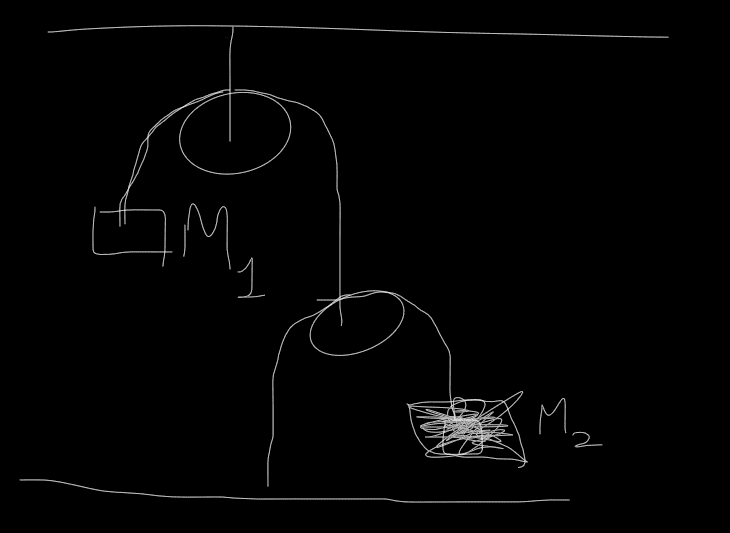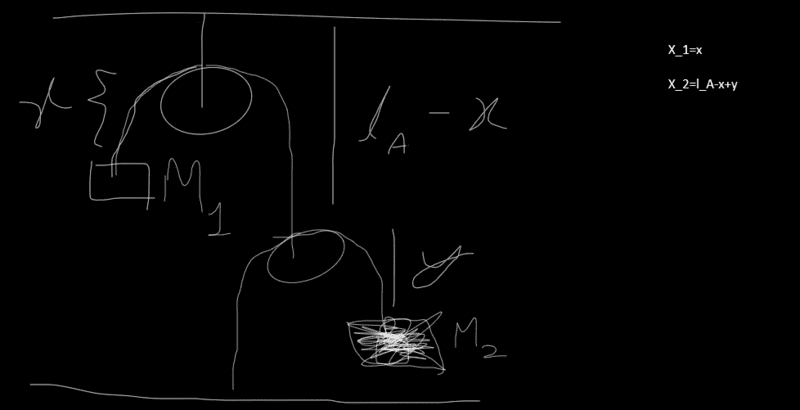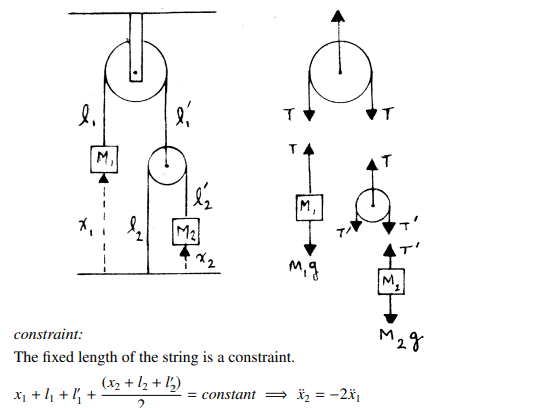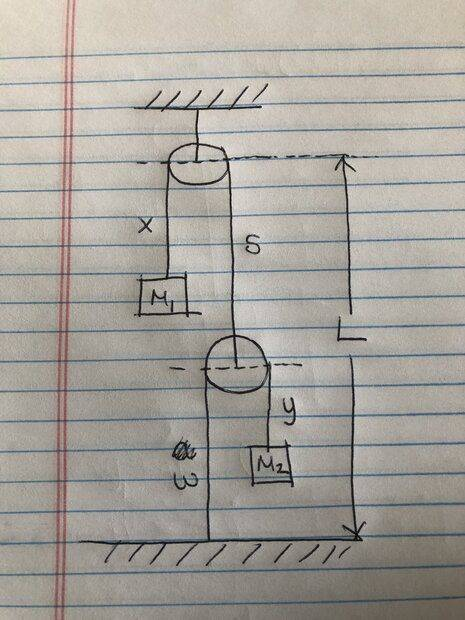# Double pulley problem using Lagrangian

• mcconnellmelany

#### mcconnellmelany

Homework Statement
The diagram of the problem is attached on the body and strings are massless. Find the acceleration of M_1
Relevant Equations
L=T-U
Euler-Lagrange equationSetting up coordinates for the problem##L=\frac{1}{2}M_1 \dot{x}^2+\frac{1}{2}M_2(\dot y-\dot x)^2+M_1gx+M_2g(l_a-x+y)##

After using Euler Lagrange for x component and y component separate and substitute one to another then I get that ##\ddot{x}=\frac{M_1-2M_2}{M_1}g##

whereas on the solution they use Newtonian method and get that ##\ddot{x}=\frac{2M_2-M_1}{4M_2+M_1}##

And I thought my method of solving the question was wrong so I tried applying M_3=0 kg on [the solution](https://youtu.be/HbmN5Xb6dYg?t=566). I still can't get the answer the book wrote.

I was trying to write constraint another way...
##2x=l_A##
##l_A-x+2y=0##
for first and second rope respectively. substituted l_A and got x=-2y.. I end up with ##\ddot{x}=\frac{2M_1-M_2}{8M_1+2M_2}##. too close. Am I applying constraint correctly?

##y=2x##

Welcome!

•Delta2
##y=2x##

Welcome!

•Delta2
What constraint did you use for the Newtonian approach? You should use the same for the Lagrangian approach too, you just can't pick different constraint in order to make the answers match.

I think the second kinetic energy term in your lagrangian is wrong, according to my opinion it should be ##\frac{1}{2}M_2(\dot x+\dot y)^2##

But you haven't tell us exactly how you measure ##y##. Do you measure y relative to the position of the second pulley (the one that the body M_2 hangs from)?

Last edited:
•Lnewqban
@haruspex maybe you want to take a look at this and offer your lights.

I was trying to write constraint another way...
##2x=l_A##
##l_A-x+2y=0##
for first and second rope respectively. substituted l_A and got x=-2y.. I end up with ##\ddot{x}=\frac{2M_1-M_2}{8M_1+2M_2}##. too close. Am I applying constraint correctly?

•Delta2
I would place the datum at the center of the pulley that holds ##M_1##.

Call the length of rope on the left ##x## as you have done, and on the right ##s##.

Then ##\dot{s} = -\dot{x}##

The kinetic energy for block ##M_2## I think is given by ## \frac{1}{2}M_2 \left( \dot s + \dot y \right)^2 = \frac{1}{2}M_2 \left( \dot y - \dot x \right)^2 ##

Then redefine all of your potential energies in from that datum

Edit:
Wait, I see that is what you have done! So I’m not sure. Should your potentials be negative?

I feel like I’m probably wrong and what @Delta2 said is correct for the kinetic energy of block 2. One seems to have ## 4 \dot x \dot y ## more kinetic energy than the other, and without using Newton’s second to confirm I’m not sure. I’m tapping out!

Last edited:
•Delta2
I took constraint differently but time derivative of position is same as the solution manual (they took second derivative for acceleration but the result is same for first order also)•Delta2
I think the second kinetic energy term in your lagrangian is wrong, according to my opinion it should be
took first time derivative of x_2 (the equation is on first picture I attached)

took first time derivative of x_2 (the equation is on first picture I attached)
Yes ok I see now.

I feel like I’m probably wrong and what @Delta2 said is correct for the kinetic energy of block 2. One seems to have 4x˙y˙ more kinetic energy than the other, and without using Newton’s second to confirm I’m not sure. I’m tapping out!
Does #10 answer your question? If it doesn't than I will say that motion of first body doesn't depend on y and potential for the body is -m1gx (but for the Lagrangian the negative becomes positive) but second body depends on both x and y.

Last edited:
##L=1/2 M1\dot x^2+1/2M2(\dot x+\dot y)^2+M1 gx-M2g\frac{x}{2}##
To shorten the Lagrangian I ignored other constant terms for second body (since they will vanish for derivative with respect to x)

Do you measure y relative to the position of the second pulley (the one that the body M_2 hangs from)?
I have written all of them in my first diagram. If I understood your question correctly then the answer is yes.

I think @haruspex wants to see your work on how you worked with the Euler-Lagrange equations for this system and how you used with them the constraint ##\dot x=-2\dot y##

I know its going to be a bit tiresome to type in Latex your exact workings, but you got to do it if you want us to pinpoint your mistake.

I think @haruspex wants to see your work on how you worked with the Euler-Lagrange equations for this system and how you used with them the constraint ##\dot x=-2\dot y##
Since I have written down the first one so I am going to write second one.
##L=\frac{1}{2}M_1\dot{x}^2+\frac{1}{2} M_2(\dot{x}-\frac{\dot x}{2})^2+M_1gx-M_2g\frac{x}{2}##
I have written first line on #14.
Second term on the above equation becomes 1/8 and last term becomes 1/2.
From Euler-Lagrange ##M_1\ddot x+\frac{1}{4}M_2\ddot x=M_1g-\frac{M_2g}{2}##

If you solve for ddot x then you will get what I just said earlier.

Btw I can confirm the Newtonian result is correct I also got it working on my own.

Btw I can confirm the Newtonian result is correct I also got it working on my own.
What's your opinion on the Lagrangian? I think I should have learned Newtonian method first.

What's your opinion on the Lagrangian? I think I should have learned Newtonian method first.
I think the Lagrangian is fine.

•mcconnellmelany
The constraint is actually ##\dot y=-2\dot x## NOT ##\dot x=-2\dot y##.

••Lnewqban and mcconnellmelany
Hmm there must be something wrong with lagrangian after all , I can't reach to the Newtonian result using this Lagrangian and the constraint ##y=c-2x\Rightarrow \dot y=-2\dot x##...

I can't reach to the Newtonian result using this Lagrangian and the constraint y=c−2x⇒y˙=−2x˙...
Sorry, are you saying that the constraint isn't working for you? But I have got the correct answer using the constraint.. Our x is the "solution manual's" x_1.

And after this I have solved some others problems on my own, thanks for the guide. (I was misreading solution manual from the beginning)

Sorry, are you saying that the constraint isn't working for you? But I have got the correct answer using the constraint.. Our x is the "solution manual's" x_1.

And after this I have solved some others problems on my own, thanks for the guide. (I was misreading solution manual from the beginning)
I know the constraint is correct so it must be the lagrangian. Btw what you doing at post #17 you seem to be using the constraint ##y=\frac{x}{2}## which is wrong.

•LnewqbanHow do you get the constraints from this datum setup? I get the following equations:

$$x + s = c \implies \dot x = - \dot s$$

$$w + y = k \implies \dot w = - \dot y$$

And

$$s + w = L \implies \dot s = -\dot w$$

So what am I missing or messing up?

•Delta2 and haruspex
##L=1/2 M1\dot x^2+1/2M2(\dot x+\dot y)^2+M1 gx-M2g\frac{x}{2}##
To shorten the Lagrangian I ignored other constant terms for second body (since they will vanish for derivative with respect to x)
That's just one equation. I asked to see your working; that means all of it.
i suspect that at some point you have become confused between the ##y## in there and in your post #1 diagrams and the ##x_2## in post #9. In their relationships to ##x## and ##x_1,## one has a factor 2, the other does not.

I managed to solve it via Lagrangian method , using as Lagrangian $$L=\frac{1}{2}M_1\dot x^2+\frac{1}{2}M_2\dot y^2-M_1gx-M_2gy$$ and the constraint $$y=c-2x\Rightarrow \dot y=-2\dot x$$ where ##c## "proper" constant...

PS. I took ##x## and ##y## as ##x_1## and ##x_2## respectively in figure of post #9, so ##y## is defined differently which allows our Lagrangian terms to have a simple form.

Last edited:
•erobz